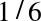# Distributions

In statistics a distribution is a set of values and their corresponding probabilities.

For example, if you roll a six-sided die, the set of possible values is the numbers 1 to 6, and the probability associated with each value is 1/6.

As another example, you might be interested in how many times each word appears in common English usage. You could build a distribution that includes each word and how many times it appears.

To represent a distribution in Python, you could use a dictionary that maps from each value to its probability. I have written a class called `Pmf` that uses a Python dictionary in exactly that way, and provides a number of useful methods. I called the class Pmf in reference to a probability mass function, which is a way to represent a distribution mathematically.

`Pmf` is defined in a Python module I wrote to accompany this book, `thinkbayes.py`. You can download it from http://thinkbayes.com/thinkbayes.py. For more information see “Working with the code”.

To use `Pmf` you can import it like this:

`from thinkbayes import Pmf`

The following code builds a Pmf to represent the distribution of outcomes for a six-sided die:

```pmf = Pmf()
for x in [1,2,3,4,5,6]:
pmf.Set(x, 1/6.0)```

`Pmf` creates an empty Pmf with no values. The `Set` method sets the probability associated with each value to.

Here’s another example that counts the number of times each ...

Get Think Bayes now with the O’Reilly learning platform.

O’Reilly members experience live online training, plus books, videos, and digital content from nearly 200 publishers.